Get instant live expert help with Excel or Google Sheets“My Excelchat expert helped me in less than 20 minutes, saving me what would have been 5 hours of work!”

Post your problem and you’ll get expert help in seconds.

Your message must be at least 40 characters
Our professional experts are available now. Your privacy is guaranteed.

How to use the delta symbol in Excel – Excelchat

Sometimes we work with symbols in Excel. In this tutorial, we will learn how to type the delta symbol, use the Insert dialog and write delta using the Autocorrect feature. We will also explore the many easy ways to insert the Delta symbol and many other symbols into our Excel worksheet.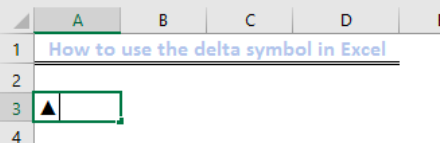Figure 1 – how to make the delta symbol in Excel

Using the delta symbol Keyboard shortcut

Typically, there’s no delta symbol on your Keyboard for inserting the Delta symbol. But this only works if we have a 10 Digit numeric keypad. If we don’t then we need to enable Num lock before taking the steps below:

• We will select the cell where we want to insert our delta symbol
• Next, we will press F2 to enter the Editing mode
• Now, we will press and hold the ALT key while typing 30 from the numeric keypad. When we release the alt key, we will find the Delta symbol.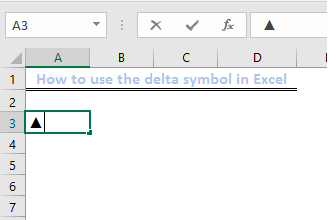Figure 2 – how to write delta

How to type delta by changing the font

• We will enter the alphabet D (in capitals) in the cell where we want the delta symbol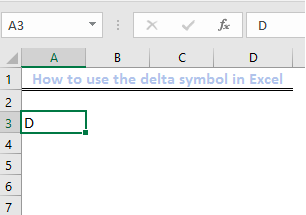Figure 3 –  how to make a delta symbol from the letter D

• Next, we will change the font style from the Home Tab to Symbol.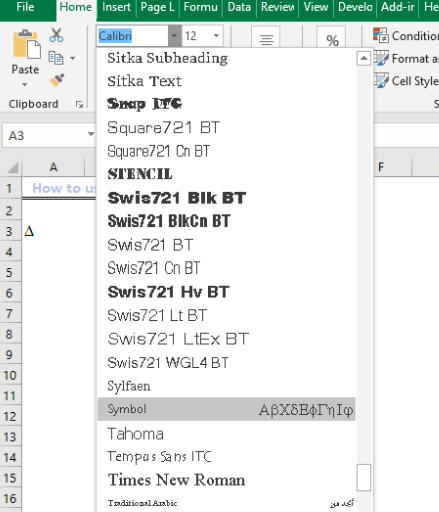Figure 4 -how to insert delta sign

Note: This quick method may not be suitable if we want to add other text in the same cell. This is because it will change the entire text in that highlighted area to symbols

Using delta Symbol Insert Dialog

• We will click on Insert Tab on the ribbon
• Next, we will select the Symbols Icon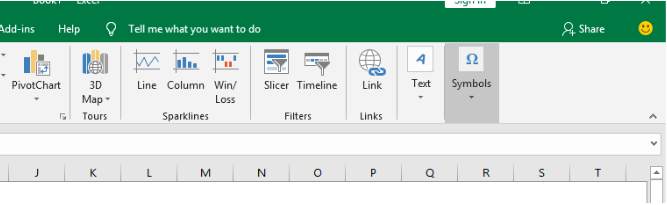Figure 5 – delta symbol shortcut

• In the Symbol dialog box, we select Greek and Coptic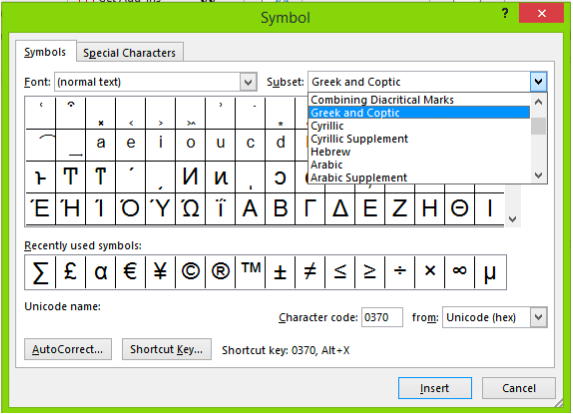Figure 6 – Using the delta character

• Here, we can scroll down and select the delta symbol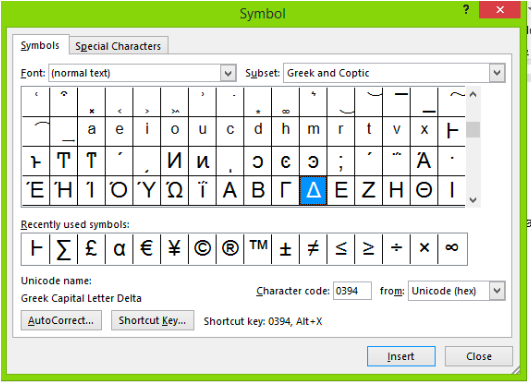Figure 7 – using a delta symbol in excel

• After which, we will click on insert

Using the Excel Autocorrect Feature

We can equally use the Excel Autocorrect feature which was specifically created to identify and create a list of codes for symbols we want. For this tutorial, we will assign DELT as code for the Delta symbol.

Here’s how to use it:

• We click on the File tab
• Next, we pick Options,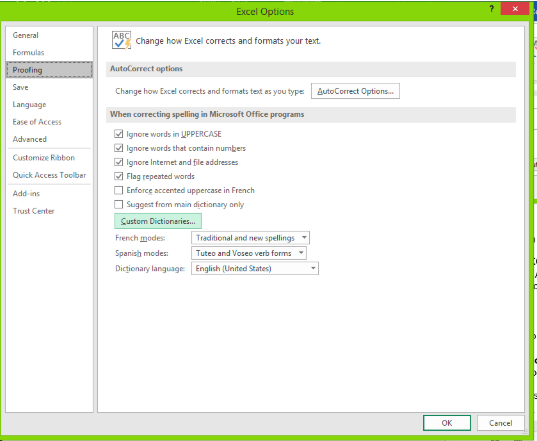Figure 8- how to use the delta sign

• then Options dialog box, pick Proofing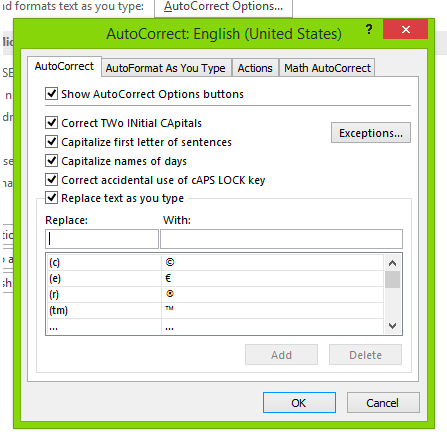Figure 9 – Using Autocorrect for delta alt code

• Then, we will select the AutoCorrect Options button
• In the AutoCorrect dialog box, we enter:
• DELT in the input box enter
• Paste the delta ( Δ) symbol in the With Input box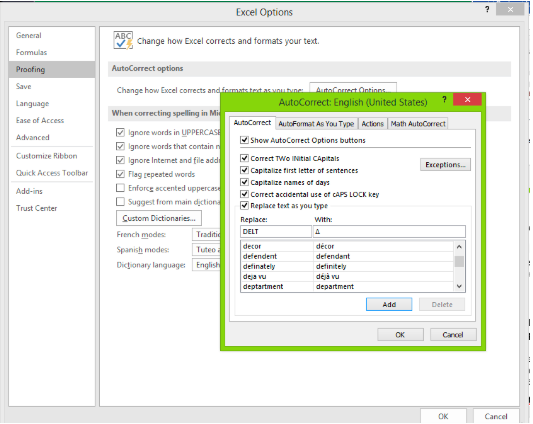Figure 10 – Using the symbol for delta

Whenever we input DELT in any Excel file, it will convert to the delta symbol.

Note: This is not also a dynamic method, because when we want to enter the symbol at any time, we need to remember our special codes

Applying Custom formatting to type delta symbol

• We will select a cell where we want to apply the custom formatting with the delta symbol
• Next, we will right click and tap the format option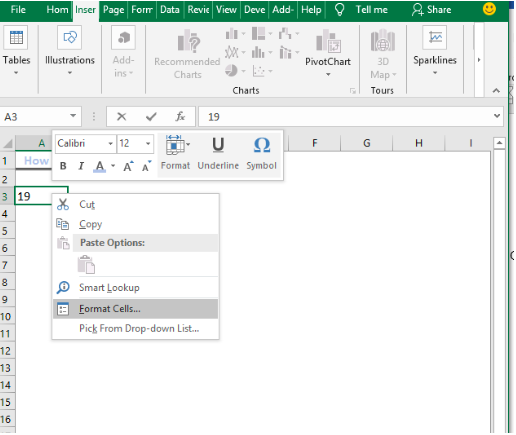Figure 11 – how to type delta

• We  will select Custom right in the Category and in the format input bar, we enter the format below and click OK

General Δ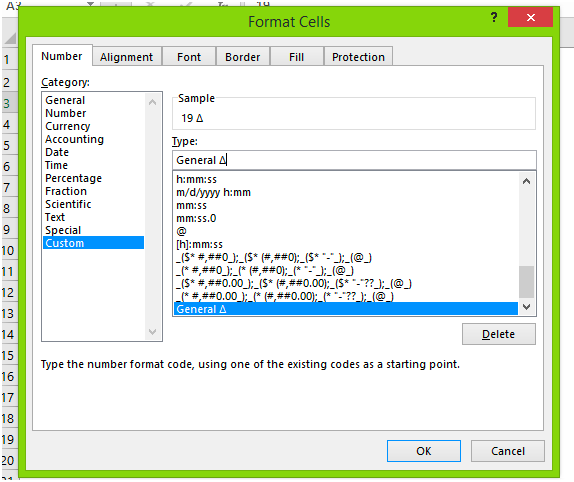Figure 12 – how to insert delta

• Now we can apply the custom format at any time to show the delta sign alongside other contents in our cell.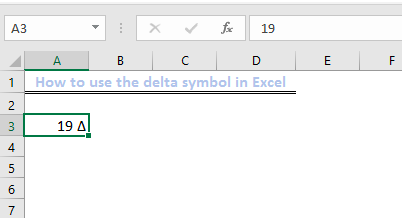Figure 13 – how to type the delta character

Note: This method only displays the Delta symbol as though it is in that cell. If we want to apply the Delta symbol for real, we may need to apply other methods.

Using Excel VBA to insert Delta symbol to multiple cells

When we want to insert Delta symbol into multiple cells, we can use the code below by following these steps

• We will go to the Developer tab and select Macro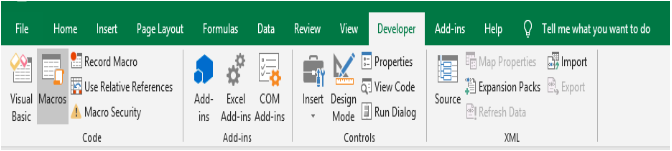Figure 14 – Using the delta symbol in Macro

• Next, we will enter our desired Macro name and select Create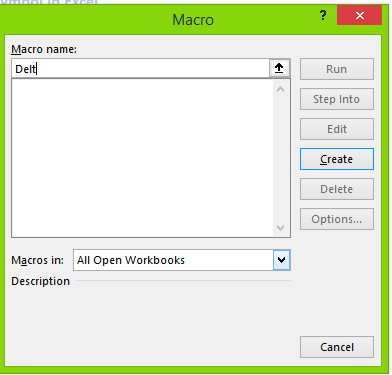Figure 15 – how to type a delta symbol

• Next, we will enter the macro below in the VBA Module that opens up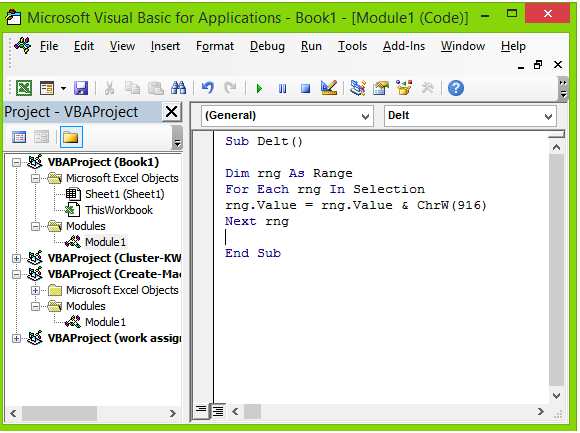Figure 16 – how to type a delta

Sub Macro1()

Dim rng As Range

For Each rng In Selection

rng.Value = rng.Value & ChrW(916)

Next rng

End Sub

Explanation

We can use this tutorial to insert all kinds of symbols. It does not have to be the Greek Delta Symbol. Another unusual way of inserting symbols is by copying them off the internet or another worksheet.

Instant Connection to an Excel Expert

Most of the time, the problem you will need to solve will be more complex than a simple application of a formula or function. If you want to save hours of research and frustration, try our live Excelchat service! Our Excel Experts are available 24/7 to answer any Excel question you may have. We guarantee a connection within 30 seconds and a customized solution within 20 minutes.